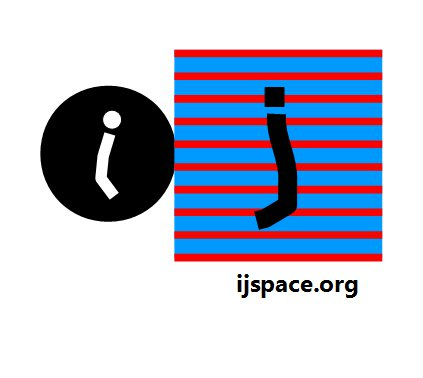1. The following correction is provided by Robert Munafo on 16/02/2014. His message is reproduced here. Thank you Robert!

The standard value of 1/alpha is 137.035999074 with an uncertainty of 44 in the last 2 digits. For your formula (e^(1-q)/(pi/2))^2 to produce this value, q would need to be about 3.00853912384, with an uncertainty of 16 in the last two digits. That's a bit bigger than 3.007...

Hypercalc output:
C1 = alpha = 1/137.035999074(44)
R1 = alpha: 0.0072973525698(23)
C2 = y = sqrt(alpha)
R2 = y: 0.085424543134(14)
C3 = x = y * pi/2
R3 = x: 0.134184558574(22)
C4 = 1-ln(x)
R4 = 3.00853912384(16

The original statement we reported on http://www.ijspace.org/Alpha/Alpha.html was,
"The exact value of the fine-structure constant corresponds to q ~ 3.007."
---------------------------------------------------------------------------------------